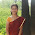# How Do Assertions Work?

```@Test
public void testScript()
{
int count = 5;
assertTrue("the count is not greater than 0", count > 0);
assertTrue(count > 0);
assertEquals("the count is not 5", count, 5);
assertEquals(count, 5);
}
```

One of the things that you will use very frequently in test automation is assertions:

1. assertTrue
2. assertEquals
3. assertFalse

They allow adding verifications to the unit tests.

If an assertion passes, the unit test execution continues.
If an assertion fails, the unit test execution stops and the remaining code lines are ignored.

If we use them so much, how about we try to understand how they work?

So, how do assertions work?

For this task, we will look at the junit framework code from the GitHub repository:
https://github.com/junit-team/junit4

The Assert.java class is the one that implements all JUNIT assertions:

We will look first at methods that implement assertTrue.

assertTrue

There are 2 methods that implement assertTrue:

1. assertTrue method that takes 2 parameters:

• condition to be evaluated
• message to be displayed in case the assertion fails

The code is so simple:

```public static void assertTrue(String message, boolean condition)
{
if (!condition)
{
fail(message);
}
}
```

If the result of evaluating the condition is false, the method fails because of the fail(message) method.

The fail(message) method has 2 versions as well:

```public static void fail(String message)
{
if (message == null)
{
throw new AssertionError();
}
throw new AssertionError(message);
}
```

The first fail(message) method checks if the message parameter is null.

If it is null, it throws an exception.
If the message is not null, it throws the same exception with the message as a parameter.

```public static void fail()
{
fail(null);
}
```

The second fail() method calls the first fail() method with null as parameter.

2. assertTrue method with 1 parameter (condition to be evaluated)

```public static void assertTrue(boolean condition)
{
assertTrue(null, condition);
}
```

This method calls the first assertTrue() method with null as message.

And this is all code needed for implementing assertTrue.

A few things need to be noticed:

• both assertTrue methods are static; using static is not a good programming practice but sometimes it is necessary
• the methods are very simple and short; one method calls the other

assertEquals

Similar with assertTrue, there are 2 methods that implement assertEquals (actually more than 2):

1. method with 3 parameters

• expected - expected value of the variable to be evaluated
• actual - actual value of the variable to be evaluated
• message - message to be displayed if expected is not equal to actual

```/*
compares expected and actual using the equalsRegardingNull() method.
the method ends if the equalsRegardingNull() result is true.
otherwise, the remaining code is executed.

the remaining code is executed if expected is different from actual.
an exception will be thrown.
if both expected and actual are strings, a ComparisonFailure exception is generated.
otherwise, the method calls failNotEquals() method which calls fail().
*/

public static void assertEquals(String message, Object expected, Object actual)
{

if (equalsRegardingNull(expected, actual))
{
return;
}

if (expected instanceof String && actual instanceof String)
{
String cleanMessage = message == null ? "" : message;
throw new ComparisonFailure(cleanMessage, (String) expected, (String) actual);
}
else
{
failNotEquals(message, expected, actual);
}

}
```
```/*
if expected and actual are both null, returns true (expected = actual).
if expected is null but actual is not, returns false (expected <> actual).
if expected is not null, it returns the result of comparing expected with actual.
*/

private static boolean equalsRegardingNull(Object expected, Object actual)
{
if (expected == null)
{
return actual == null;
}
return isEquals(expected, actual);
}
```
```/*
if expected is equal to actual, it returns true.
if expected is not equals to actual, it returns false.
*/

private static boolean isEquals(Object expected, Object actual)
{
return expected.equals(actual);
}

private static void failNotEquals(String message, Object expected, Object actual)
{
fail(format(message, expected, actual));
}
```

2. method with 2 parameters (expected and actual values of the variable to be evaluated)

This method calls the assertEquals method with 3 parameters and makes the first parameter null.

```public static void assertEquals(Object expected, Object actual)
{
assertEquals(null, expected, actual);
}
```

So, what can we learn from this code?

• One of the best sources of learning how something works is the source code
• If we want to learn how to code better, the source code of popular frameworks provides many good lessons. In this case, the code uses many small methods which are very simple and clear instead of bigger methods.
• Use custom exceptions for making failures visible

1.